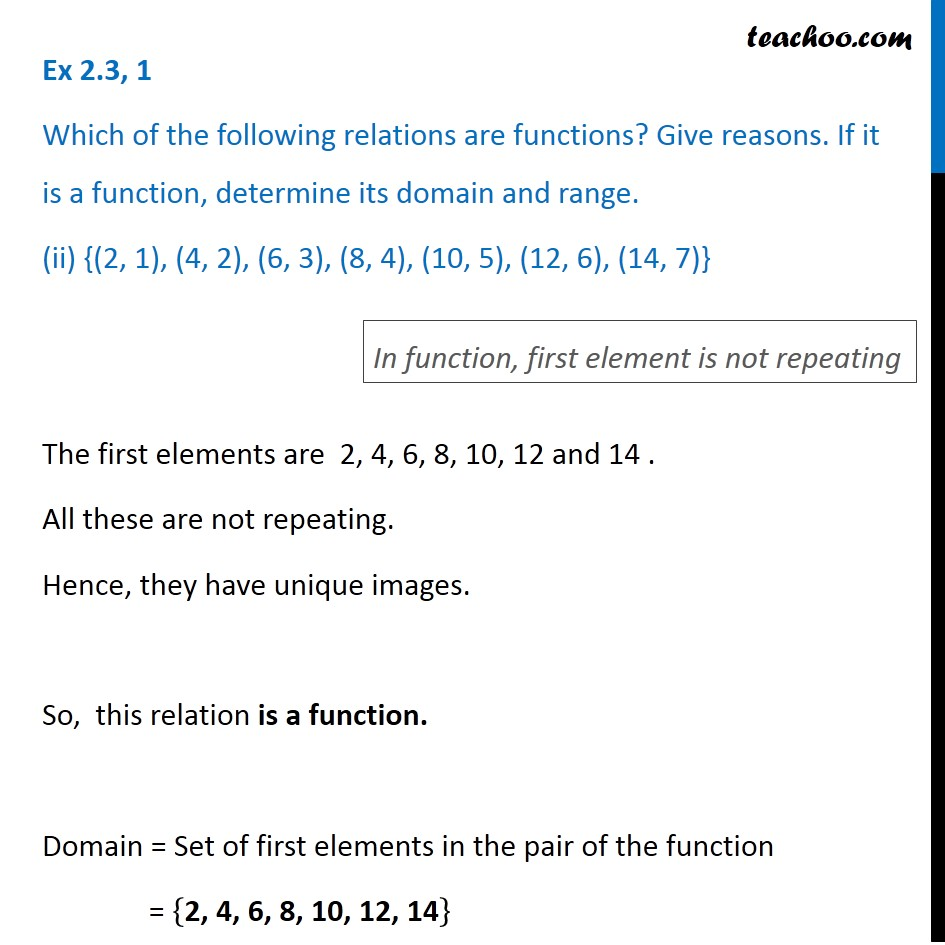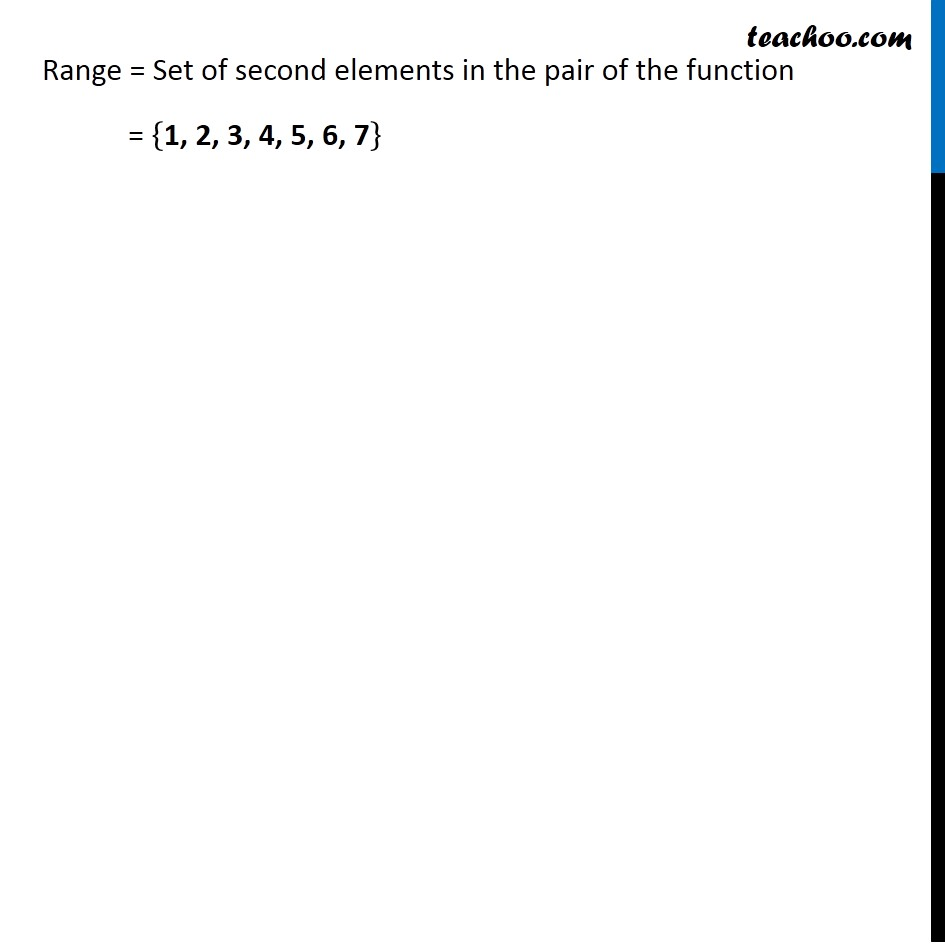1. Chapter 2 Class 11 Relations and Functions (Term 1)
2. Serial order wise
3. Ex 2.3

Transcript

Ex 2.3, 1 Which of the following relations are functions? Give reasons. If it is a function, determine its domain and range. (ii) {(2, 1), (4, 2), (6, 3), (8, 4), (10, 5), (12, 6), (14, 7)} The first elements are 2, 4, 6, 8, 10, 12 and 14 . All these are not repeating. Hence, they have unique images. So, this relation is a function. Domain = Set of first elements in the pair of the function = {"2, 4, 6, 8, 10, 12, 14" } In function, first element is not repeating Range = Set of second elements in the pair of the function = {"1, 2, 3, 4, 5, 6, 7" }